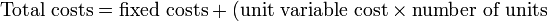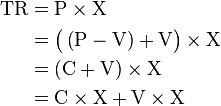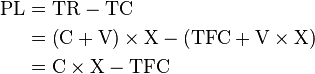Cost Volume Profit analysis is the analysis which is done on the basis of Break Even Point Analysis. It is used for getting target operating income. Before explaining CVP Analysis deeply, I try to explain the meaning of Cost Volume Profit. Cost Volume Profit is the point where total cost is equal to total revenue.

In simple words, CVP is the second name of break even point. We know there is big relationship between cost and our profit or revenue. If we fix this break even point, it will be very easy for us to calculate target operating income or revenue by getting formula from simple equation of total revenue = total cost.

Total Revenue = Sale price  X quantity sold

TR = P X X units sold

Total Cost = Fixed Cost + Variable Cost$\text{Total costs} = \text{fixed costs} + (\text{unit variable cost} \times \text{number of units}$)where
• C = Unit Contribution (Margin)
• TC = Total costs
• TFC = Total fixed costs
• V = Unit variable cost (variable cost per unit)
• X = Number of units
• TR = S = Total revenue = Sales
• P = (Unit) sales price

Subtracting variable costs from both costs and sales yields the simplified diagram and equation for profit and loss.
In symbols:\begin{align} \text{PL} &= \text{TR} - \text{TC}\\ &= \left(\text{C}+\text{V}\right)\times \text{X} - \left(\text{TFC} + \text{V} \times \text{X}\right)\\ &= \text{C} \times \text{X} - \text{TFC} \end{align}

or

Profit Level = Contribution per unit X units sold - Total Fixed Cost

Or

( Profit level + Total Fixed Cost ) / Contribution per unit = Unit Sold

Cost Volume Profit Analysis is used for maximize the profit of company. In this analysis, we divide the total cost into fixed cost and variable cost. Fixed cost will not change at any level of production. But, only variable cost will change at different level of production. Now, company will change the level of production due to increasing of demand of products or effective use of resources or increasing in the selling price of products. So, to check its effect on the profit, we need to analyze cost volume profit.

In this analysis, we fix the break even point's quantity through chart and try to change the level of production after this break even point level quantity for maximize the profit and minimize the loss.

Cost Volume Analysis will give the Answer of Following Questions

Q:- 1. How many Quantity  must be sold?

Assume that the Pants Shop  variable costs amount to $42 per unit. The average selling price per pair of pants is$70 and total fixed costs amount to $84,000. In this example, we will calculate the contribution margin first which is$ 70 - $42 =$ 28 per unit, then

If company has to achieve the net profit of $14000, he has to sell the quantity on the basis of the formula ( Profit level + Total Fixed Cost ) / Contribution per unit = Unit Sold Q :- 2. What dollar sales are needed to achieve this income? Now, if businessman wants to know the value of sales, then, he only divide (total fixed cost+ net profit) with contribution margin %. Contribution margin% = Contribution per unit / selling price per unit X 100 =$28 ÷ $70 = 40% Except above two questions, CVP analysis is helpful for decision making in many other business problems like Q:- 3. Should the business advertise? If the management anticipates selling 3,200 pairs of pants. Management is considering an advertising campaign that would cost$10,000.  It is anticipated that the advertising will increase sales to 4,000 units.

We are seeing that operating profit is increasing with highest rate, so, we should advertise.

Q:- 4. Should management decrease the selling  price per pair of pants to $61? Instead of advertising, management is considering reducing the selling price to$61 per pair of pants. It is anticipated that this will increase sales to 4,500 units.

Still increasing of quantity sold, our operating income is just $1500 which is very low due to decreasing the sale price, so, no need to take such decision.. ##$type=three$a=hide$cm=hide$s=hide$show=/2019/08/how-to-spend-money-intelligently.html$l=hide$c=9$d= ##$type=three$a=hide$cm=hide$s=hide$show=/2019/08/how-to-buy-gold-from-paytm.html$l=hide$c=6$d=no ##$type=three$a=hide$cm=hide$s=hide$show=/2013/06/tdr-vs-stdr.html$l=hide$c=30$d=no ##$type=three$a=hide$cm=hide$s=hide$show=/2019/06/10-steps-to-become-better-investor.html\$l=hide

Name

false
ltr
item
Accounting Education: Cost Volume Profit Analysis
Cost Volume Profit Analysis
http://1.bp.blogspot.com/-lNRlUutLfb0/Ug16DcLjkII/AAAAAAAAL5o/kTP_er13GPw/s400/cvp+5.PNG
http://1.bp.blogspot.com/-lNRlUutLfb0/Ug16DcLjkII/AAAAAAAAL5o/kTP_er13GPw/s72-c/cvp+5.PNG
Accounting Education
https://www.svtuition.org/2013/08/cost-volume-profit-analysis.html
https://www.svtuition.org/
http://www.svtuition.org/
http://www.svtuition.org/2013/08/cost-volume-profit-analysis.html
true
2410664366776677676
UTF-8